# 「建议收藏」送你一份精心总结的3万字ES6实用指南（下）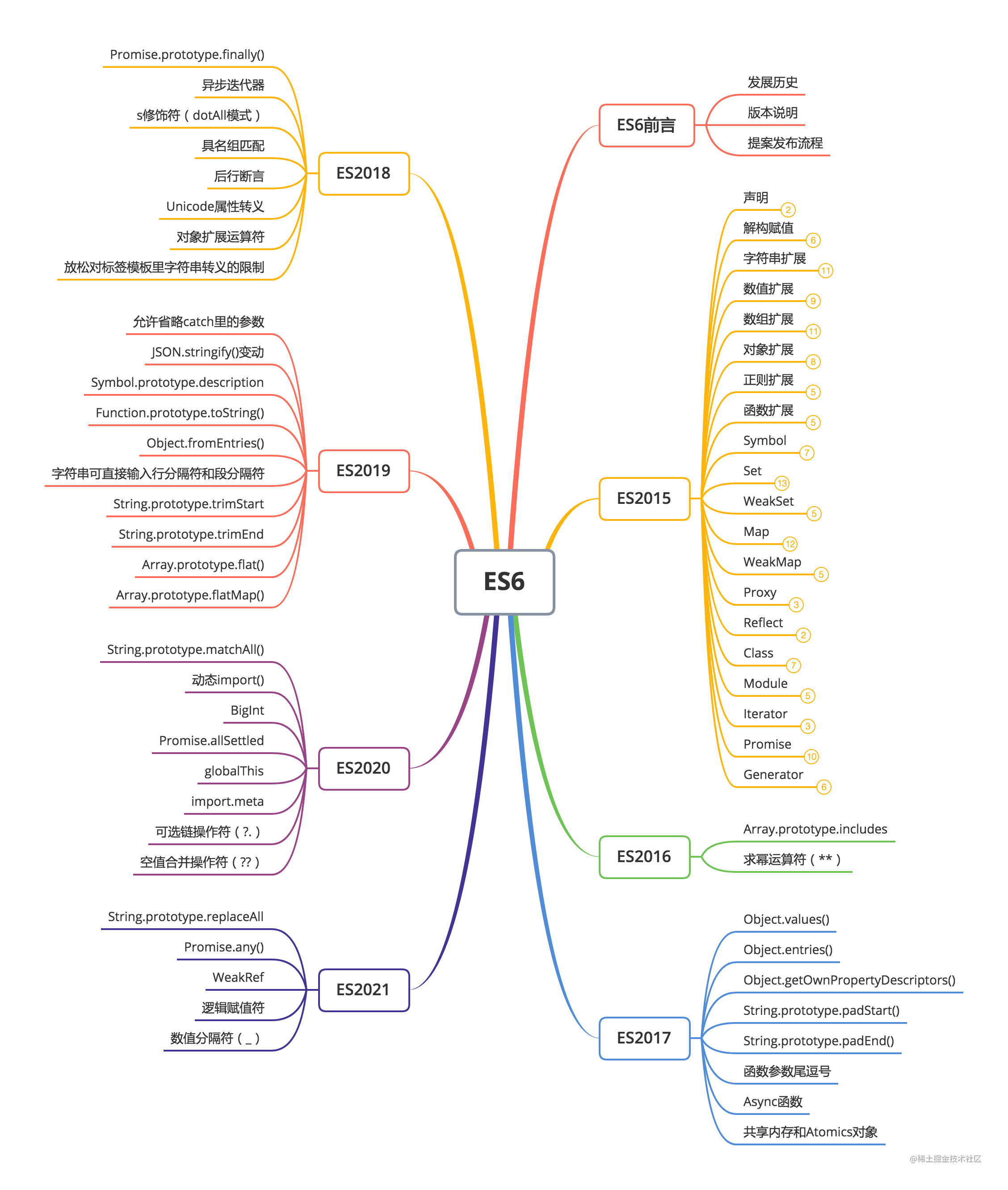## ES2016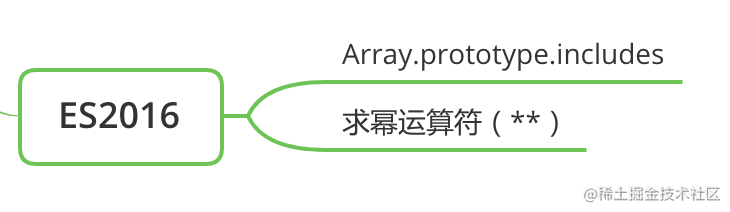### Array.prototype.includes

if (arr.indexOf(el) >= 0) {}

// 或者
if (~arr.indexOf(el)) {}

if (arr.includes(el)) {}

indexOf 会返回找到元素在数组中的索引位置，判断的逻辑是是否严格相等，所以他在遇到 NaN 的时候不能正确返回索引，但是 includes 解决了这个问题：

[1, NaN, 3].indexOf(NaN)   // -1
[1, NaN, 3].includes(NaN)  // true

### 求幂运算符（**）

x ** y 是求 xy 次幂，和 Math.pow(x, y) 功能一致：

// x ** y
let squared = 2 ** 2  // 2 * 2 = 4
let cubed = 2 ** 3    // 2 * 2 * 2 = 8

x **= y 表示求 xy 次幂，并且把结果赋值给 x

// x **= y
let x = 2;
x **= 3  // x 最后等于 8

## ES2017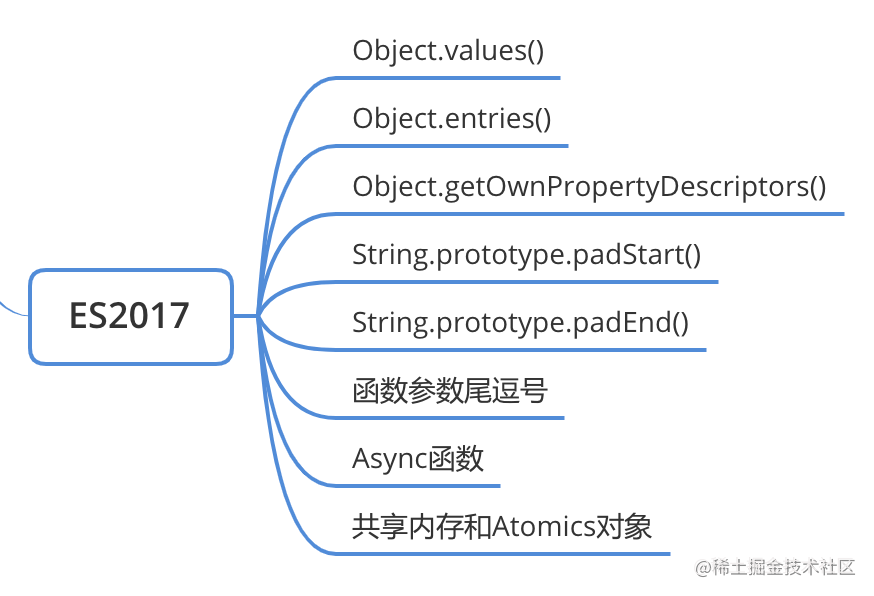### Object.values()

const person = { name: '布兰' };
Object.defineProperty(person, 'age', {
value: 12,
enumrable: false  // age 属性将不可遍历
})
console.log(Object.values(person))  // ['布兰']

// 类似 str.split('') 效果
console.log(Object.values('abc'))  // ['a', 'b', 'c']

### Object.entries()

const person = { name: '布兰', age: 12 }
console.log(Object.entries(person))  // [["name", "布兰"], ["age", 12]]

const person = { name: '布兰', age: 12 }
const map = new Map(Object.entries(person))
console.log(map)  // Map { name: '布兰', age: 12 }

### Object.getOwnPropertyDescriptors()

Object.getOwnPropertyDescriptor() 会返回指定对象某个自身属性的的描述对象，而 Object.getOwnPropertyDescriptors() 则是返回指定对象自身所有属性的描述对象：

const person = { name: '布兰', age: 12 }

console.log(Object.getOwnPropertyDescriptor(person, 'name'))
// { configurable: true, enumerable: true, value: "布兰", writable: true }

console.log(Object.getOwnPropertyDescriptors(person))
//{
//  name: { configurable: true, enumerable: true, value: "布兰", writable: true },
//  age: {configurable: false, enumerable: false, value: 12, writable: false}
//}

const shallowClone = (obj) => Object.create(
Object.getPrototypeOf(obj),
Object.getOwnPropertyDescriptors(obj)
)

str.padStart(length [, padStr]) 会返回一个新字符串，该字符串将从 str 字符串的左侧开始填充某个字符串 padStr（非必填，如果不是字符串则会转成字符串, 传入 undefined 和不传这个参数效果一致）直到达到指定位数 length 为止：

'abc'.padStart(5, 2)          // '22abc'

'abc'.padEnd(5, 2)  // 'abc22'

### 函数参数尾逗号

function init(
param1,
param2,
) { }

init('a', 'b',)

### Async函数

• 使用 async 可以声明一个 async 函数，结合 await 可以用一种很简介的方法写成基于 Promise 的异步行为，而不需要刻意的链式调用。await 表达式会暂停整个 async 函数的执行进程并出让其控制权，只有当其等待的基于 Promise 的异步操作被兑现或被拒绝之后才会恢复进程。async 函数有如下几种定义形式：

// 函数声明
async function foo() {}

// 函数表达式
let foo = async function() {}

// 箭头函数
let foo = async () => {}

// 对象方法
lef obj = {
async foo() {}
}

// 类方法
class Dog {
async bark() {}
}
复制代码
• async 函数一定会返回一个 Promise 对象，所以它可以使用 then 添加处理函数。如果一个 async 函数的返回值看起来不是Promise，那么它将会被隐式地包装在一个 Promise 中：

async function foo() {
return 'a'
}
foo().then(res => {
console.log(res)  // 'a'
})
复制代码
• 内部如果发生错误，或者显示抛出错误，那么 async 函数会返回一个 rejected 状态的 Promsie

async function foo() {
throw new Error('error')
}
foo().catch(err => {
console.log(err)  // Error: error
})
复制代码
• 返回的 Promise 对象必须等到内部所有 await 命令 Promise 对象执行完才会发生状态改变，除非遇到 return 语句或抛出错误；任何一个 await 命令返回的 Promise 对象变 为rejected 状态，整个 Async 函数都会中断后续执行：

async function fn() {
let a = await Promise.resolve('success')
console.log('a_' + a)
let b = await Promise.reject('fail')
console.log('b_' + b)  // 不会执行
}
fn().then(res => {
console.log(res)  // 不会执行
}, err => {
console.log(err)
})
// 'a_success'
// 'fail'
复制代码
• 所以为了保证 async 里的异步操作都能完成，我们需要将他们放到 try...catch() 块里或者在 await 返回的 Promise 后跟一个 catch 处理函数：

async function fn() {
try {
let a = await Promise.reject('a fail')
console.log('a_' + a)  // 不会执行
} catch (e) {
console.log(e)  // 'a fail'
}
let b = await Promise.reject('b fail')
.catch(e => {
console.log(e)  // 'b fail'
})
console.log('b_' + b)  // 'bundefined'
}
fn().then(res => {
console.log(res)  // undefined
}, err => {
console.log(err)  // 不会执行
})
复制代码
• 如果 async 函数里的多个异步操作之间没有依赖关系，建议将他们写到一起减少执行时间：

// 写法一
let [foo, bar] = await Promise.all([getFoo(), getBar()])

// 写法二
let fooPromise = getFoo()
let barPromise = getBar()
let foo = await fooPromise
let bar = await barPromise
复制代码
• await 命令只能用在 async 函数之中，如果用在普通函数，就会报错。

## ES2018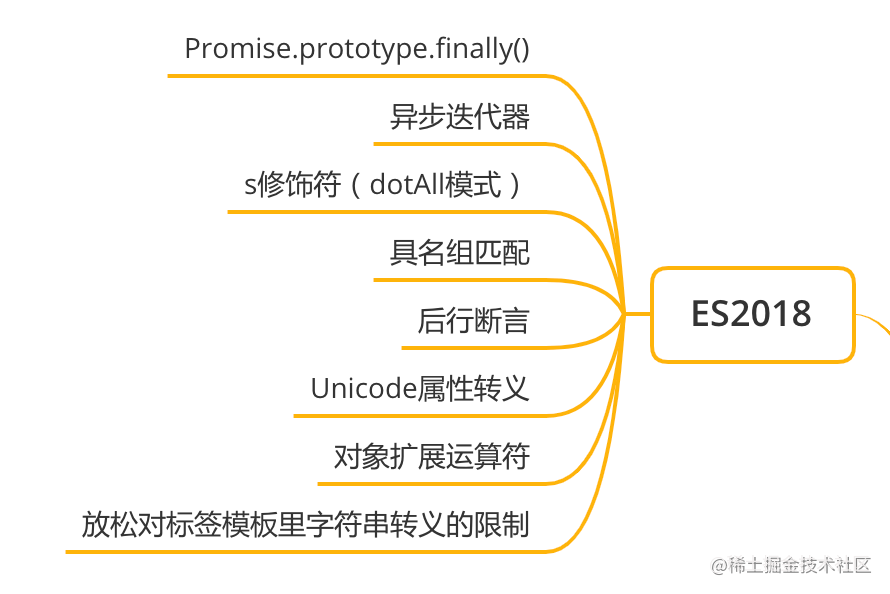### Promise.prototype.finally()

Promise.prototype.finally() 用于给 Promise 对象添加 onFinally 函数，这个函数主要是做一些清理的工作，只有状态变化的时候才会执行该 onFinally 函数。

function onFinally() {
console.log(888)  // 并不会执行
}
new Promise((resolve, reject) => {

}).finally(onFinally)

finally() 会生成一个 Promise 新实例，finally 一般会原样后传父 Promise，无论父级实例是什么状态：

let p1 = new Promise(() => {})
let p2 = p1.finally(() => {})
setTimeout(console.log, 0, p2)  // Promise {<pending>}

let p3 = new Promise((resolve, reject) => {
resolve(3)
})
let p4 = p3.finally(() => {})
setTimeout(console.log, 0, p3)  // Promise {<fulfilled>: 3}

let p1 = new Promise((resolve, reject) => {
resolve(3)
})
let p2 = p1.finally(() => new Promise(() => {}))
setTimeout(console.log, 0, p2)  // Promise {<pending>}

let p3 = p1.finally(() => Promise.reject(6))
setTimeout(console.log, 0, p3)  // Promise {<rejected>: 6}

let p4 = p1.finally(() => {
throw new Error('error')
})
setTimeout(console.log, 0, p4)  // Promise {<rejected>: Error: error}

### 异步迭代器

let iterator = [1, 2][Symbol.iterator]()
iterator.next()  // { value: 1, done: false }
iterator.next()  // { value: 2, done: false }
iterator.next()  // { value: undefinde, done: true }

class Emitter {
constructor(iterable) {
this.data = iterable
}
[Symbol.asyncIterator]() {
let length = this.data.length,
index = 0;

return {
next:() => {
const done = index >= length
const value = !done ? this.data[index++] : undefined
return new Promise((resolve, reject) => {
resolve({value, done})
})
}
}
}
}

let emitter = new Emitter([1, 2, 3])
let asyncIterator = emitter[Symbol.asyncIterator]()
asyncIterator.next().then(res => {
console.log(res)  // { value: 1, done: false }
})
asyncIterator.next().then(res => {
console.log(res)  // { value: 2, done: false }
})
asyncIterator.next().then(res => {
console.log(res)  // { value: 3, done: false }
})

let asyncIterable = new Emitter([1, 2, 3])
async function asyncCount() {
for await (const x of asyncIterable ) {
console.log(x)
}
}
asyncCount()
// 1 2 3

class Emitter {
constructor(iterable) {
this.data = iterable
}
async *[Symbol.asyncIterator]() {
let length = this.data.length,
index = 0;

while (index < length) {
yield this.data[index++]
}
}
}
async function asyncCount() {
let emitter = new Emitter([1, 2, 3])
const asyncIterable = emitter[Symbol.asyncIterator]()
for await (const x of asyncIterable ) {
console.log(x)
}
}
asyncCount()
// 1 2 3

### s修饰符(dotAll模式)

/foo.bar/.test('foo\nbar')   // false
/foo.bar/s.test('foo\nbar')  // true

/foo.bar/s.dotAll  // true

### 具名组匹配

let re = /(\d{4})-(\d{2})-(\d{2})/
let result = re.exec('2015-01-02')
// result === '2015-01-02'
// result === '2015'
// result === '01'
// result === '02'

let re = /(?<year>\d{4})-(?<month>\d{2})-(?<day>\d{2})/
let result = re.exec('2015-01-02')
// result.groups.year === '2015'
// result.groups.month === '01'
// result.groups.day === '02'

let {groups: {year, month, day}} = /(?<year>\d{4})-(?<month>\d{2})-(?<day>\d{2})/.exec('2015-01-02')
console.log(year, month, day)  // 2015 01 02

// result === '02/01/2015'

### 后行断言

/(?<=\$)\d+/.exec('I have$100.')  // ['100']

/(?<!\$)\d+/.exec('I have$100.')  // ['00']

### Unicode属性转义

/\p{Emoji}+/u.exec('😁😭笑死我了🤣😂不行了')  // ['😁😭']
/\P{Emoji}+/u.exec('😁😭笑死我了🤣😂不行了')  // ['笑死我了']

### 对象扩展运算符

let {x, ...y} = {x: 1, a: 2, b: 3}
console.log(y)  // {a: 2, b: 3}

let obj = { x: 1 }
obj.__proto__ = { y: 2 }
let {...a} = obj
console.log(a.y)  // undefined

let person = Object.create({ name: '布兰' })
person.age = 12

// 浅拷贝写法一
let { ...pClone1 } = person
console.log(pClone1)  // { age: 12 }
console.log(pClone1.name)  // undefined

// 浅拷贝写法二
let pClone2 = {...person}
console.log(pClone2)  // { age: 12 }
console.log(pClone2.name)  // undefined

let ab = {...a, ...b}

// 等同于
let ab = Object.assign({}, a, b);

let aWithOverrides = { ...a, x: 1, y: 2 };

let aWithDefaults = { x: 1, y: 2, ...a };

function baseFunction({ a, b }) {}
function wrapperFunction({ x, y, ...restConfig }) {
// 使用 x 和 y 参数进行操作
// 其余参数传给原始函数
return baseFunction(restConfig)
}

ECMAScript 6 入门

## ES2019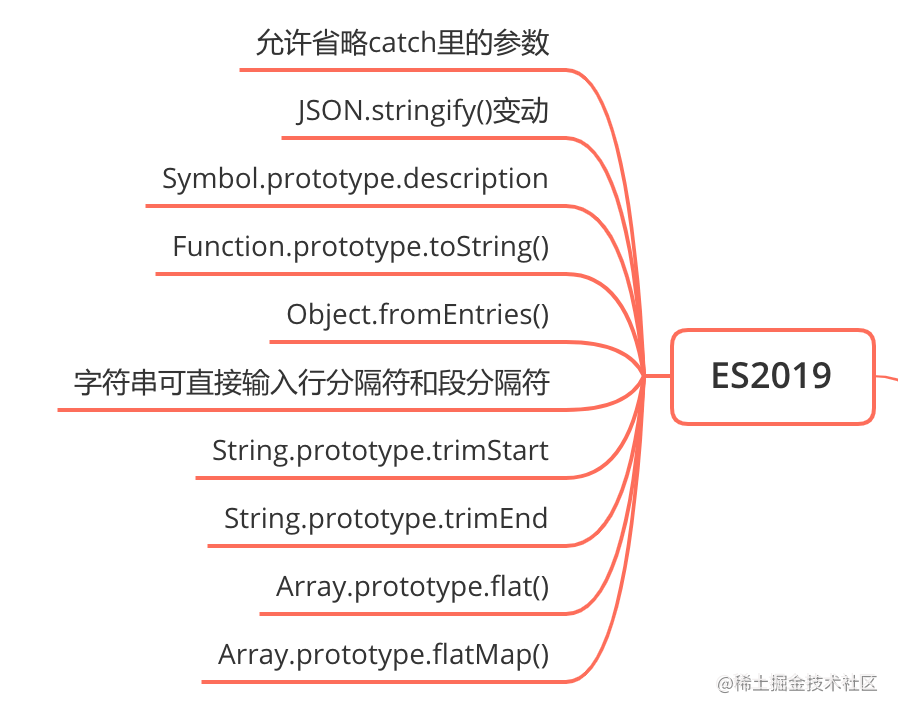### 允许省略catch里的参数

try {

} catch {

}

### JSON.stringify()变动

UTF-8 标准规定，0xD8000xDFFF 之间的码点，不能单独使用，必须配对使用。 所以 JSON.stringify() 对单个码点进行操作，如果码点符合 UTF-8 标准，则会返回对应的字符，否则会返回对应的码点：

JSON.stringify('\u{1f600}')  // ""😀""
JSON.stringify('\u{D834}')  // ""\ud834""

### Symbol.prototype.description

Symbol 实例新增了一个描述属性 description

let symbol = Symbol('foo')
symbol.description  // 'foo'

### Object.fromEntries()

Object.fromEntries() 方法是 Object.entries() 的逆操作，用于将一个键值对数组转为对象：

let person = { name: '布兰', age: 12 }
let keyValueArr = Object.entries(person)   // [['name', '布兰'], ['age', 12]]
let obj = Object.fromEntries(arr)  // { name: '布兰', age: 12 }

let person = { name: '布兰', age: 12 }

// 对象 -> 键值对数组
let keyValueArr = Object.entries(person)  // [['name', '布兰'], ['age', 12]]

// 键值对数组 -> Map
let map = new Map(keyValueArr)  // Map {"name": "布兰", "age": 12}

// Map -> 键值对数组
let arr = Array.from(map)  // [['name', '布兰'], ['age', 12]]

// 键值对数组 -> 对象
let obj = Array.from(arr).reduce((acc, [ key, val ]) => Object.assign(acc, { [key]: val }), {})  // { name: '布兰', age: 12 }

### 字符串可直接输入行分隔符和段分隔符

JavaScript 规定有 5 个字符，不能在字符串里面直接使用，只能使用转义形式。

• U+005C：反斜杠（reverse solidus)
• U+000D：回车（carriage return）
• U+2028：行分隔符（line separator）
• U+2029：段分隔符（paragraph separator）
• U+000A：换行符（line feed）

JSON.parse('"\u2028"')  // ""
JSON.parse('"\u2029"')  // ""
JSON.parse('"\u005C"')  // SyntaxError

### String.prototype.trimStart

let str = '  hello world '
let newStr = str.trimStart()
console.log(newStr, newStr === str)
// 'hello world '  false

### String.prototype.trimEnd

let str = '  hello world '
let newStr = str.trimEnd()
console.log(newStr, newStr === str)
// '  hello world'  false

### Array.prototype.flat()

arr.flat(depth) 按照 depth （不传值的话默认是 1）深度拍平一个数组，并且将结果以新数组形式返回：

// depth 默认是 1
const arr1 = [1, 2, [3, 4]]
console.log(arr1.flat())  // [1, 2, 3, 4]

// 使用 Infinity，可展开任意深度的嵌套数组；自动跳过空数组；
const arr2 = [1, , [2, [3, ]]]
console.log(arr2.flat(Infinity))
// [1, 2, 3, 4]

reduce 实现拍平一层数组：


const arr = [1, 2, [3, 4]]

// 方法一
let newStr = arr.reduce((acc, cur) => acc.concat(cur), [])

// 方法二
const flattened = arr => [].concat(...arr)
flattened(arr)

### Array.prototype.flatMap()

flatMap(callback) 使用映射函数 callback 映射每个元素，callback 每次的返回值组成一个数组，并且将这个数组执行类似 arr.flat(1) 的操作进行拍平一层后最后返回结果：

const arr1 = [1, 2, 3, 4]

arr1.flatMap(x => [x * 2])
// 将 [, , , ] 数组拍平一层得到最终结果：[2, 4, 6, 8]

## ES2020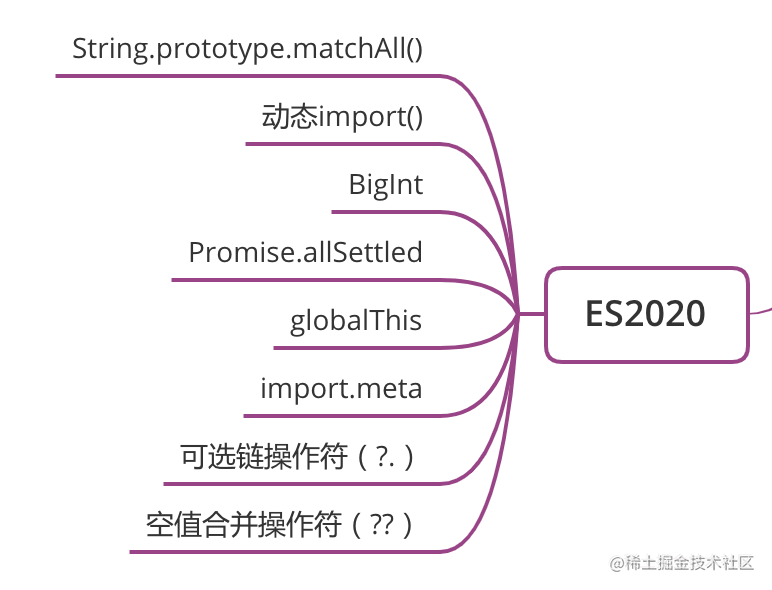### String.prototype.matchAll()

String.prototype.matchAll() 方法，可以一次性取出所有匹配。不过，它返回的是一个 RegExpStringIterator 迭代器同是也是一个可迭代的数据结构，所以可以通过 for...of 进行迭代：

let str = 'test1test2'
let regexp = /t(e)(st(\d?))/g
let iterable = str.matchAll(regexp)
for (const x of iterable) {
console.log(x)
}
// ['test1', 'e', 'st1', '1', index: 0, input: 'test1test1', groups: undefined]
// ['test2', 'e', 'st2', '2', index: 5, input: 'test1test2', groups: undefined]

// 方法一
[...str.matchAll(regexp)]

// 方法二
Array.from(str.matchAll(regexp))

### 动态import()

if (xxx) {
import('./module.js')
}

import() 是异步导入的，结果会返回一个 Promise

import('/module.js')
.then((module) => {
// Do something with the module.
})

### BigInt

BigInt 是一种内置对象，它提供了一种方法来表示大于 $2^{53}$ - 1 的整数。这原本是 Javascript 中可以用 Number 表示的最大数字。BigInt 可以表示任意大的整数。

const num1 = 10n
const num2 = BigInt(20)

NumberBigInt 之间能进行比较，但他们之间是宽松相等；且由于他们表示的是不同类型的数字，所以不能直接进行四则运算：

10n == 10         // true
10n === 10        // false
10n > 8           // true
10 + Number(10n)  // 20
10 + 10n          // TypeError

### Promise.allSettled

Promise.allSettled(iterable) 当所有的实例都已经 settled，即状态变化过了，那么将返回一个新实例，该新实例的内部值是由所有实例的值和状态组合成的数组，数组的每项是由每个实例的状态和内部值组成的对象。

function init(){
return 3
}
let p1 = Promise.allSettled([
new Promise((resolve, reject) => {
resolve(9)
}).then(res => {}),
new Promise((resolve, reject) => {
reject(6)
}),
init()
])
let p2 = p1.then(res => {
console.log(res)
}, err => {
console.log(err)
})
// [
//      {status: "fulfilled", value: undefined},
//      {status: "rejected", reason: 6},
//      {status: "fulfilled", value: 3}
// ]

### globalThis

// 在浏览器中
globalThis === window  // true

### import.meta

import.meta 是一个给 JavaScript 模块暴露特定上下文的元数据属性的对象。它包含了这个模块的信息，比如说这个模块的 URLimport.meta 必须在一个模块里使用：

// 没有声明 type="module"，就使用 import.meta 会报错
<script type="module" src="./js/module.js"></script>

// 在module.js里
console.log(import.meta)
// {url: "http://localhost/3ag/js/module.js"}

module.exports = {
chainWebpack: config => {
config.module
.rule('js')
.test(/\.js\$/)
.end()
}
}

### 可选链操作符（?.）

let obj = {
first: {
second: '布兰'
}
}

// 写法一
let name1 = ''
if (obj) {
if (obj.first) {
name1 = obj.first.second
}
}

// 写法二
let name2 = obj && obj.first && obj.first.second

?. 操作符允许读取位于连接对象链深处的属性的值，而不必明确验证链中的每个引用是否有效。如果某个属性为 null 或者 undefined 则结果直接为 undefined。有了可选链操作符就可以使得表达式更加简明了，对于上面例子用可选链操作符可以这么写：

let name3 = obj?.first?.second

### 空值合并操作符（??）

let name = '' || '布兰'
console.log(name)  // '布兰'

?? 操作符就是为了解决这个问题而出现的，x ?? y 只有左侧的操作数为 nullundefined 的时候才取右侧操作数，否则取左侧操作数：

let num = 0 ?? 1
console.log(num)  // 0

## ES2021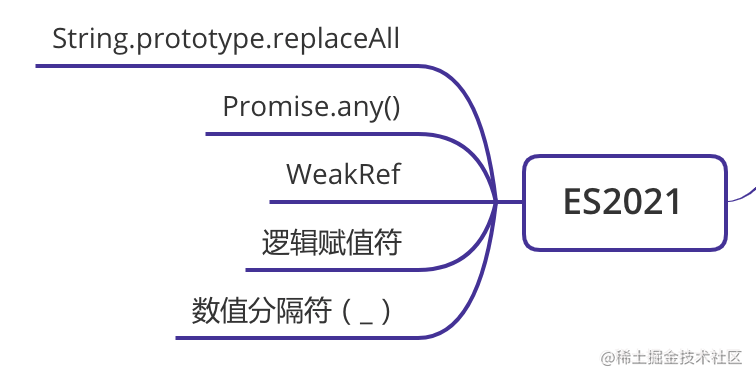### String.prototype.replaceAll

const queryString = 'q=query+string+parameters'

// 方法一
const withSpaces1 = queryString.replace(/\+/g, ' ')

// 方法二
const withSpaces2 = queryString.split('+').join(' ')

const withSpace3 = queryString.replaceAll('+', ' ')

replaceAll 的第一个参数可以是字符串也可以是正则表达式，当是正则表达式的时候，必须加上全局修饰符 /g，否则报错。

### Promise.any()

Promsie.any()Promise.all() 一样接受一个可迭代的对象，然后依据不同的入参会返回不同的新实例：

• 传一个空的可迭代对象或者可迭代对象所有 Promise 都是 rejected 状态的，则会抛出一个 AggregateError 类型的错误，同时返回一个 rejected 状态的新实例：

let p1 = Promise.any([])
let p2.catch(err => {})
setTimeout(console.log, 0, p1)
// Promise {<rejected>: AggregateError: All promises were rejected}
复制代码
• 只要可迭代对象里包含任何一个 fulfilled 状态的 Promise，则会返回第一个 fulfilled 的实例，并且以它的值作为新实例的值：

let p = Promise.any([
1,
Promise.reject(2),
new Promise((resolve, reject) => {}),
Promise.resolve(3),
])
setTimeout(console.log, 0, p)
// Promise {<fulfilled>: 1}
复制代码
• 其他情况下，都会返回一个 pending 状态的实例：

let p = Promise.any([
Promise.reject(2),
Promise.reject(3),
new Promise((resolve, reject) => {}),
])
setTimeout(console.log, 0, p)
// Promise {<pending>: undefined}
复制代码

### WeakRef

WeakRef 对象允许你保留对另一个对象的弱引用，而不会阻止被弱引用的对象被 GC 回收。WeakRef 的实例方法 deref() 可以返回当前实例的 WeakRef 对象所绑定的 target 对象，如果该 target 对象已被 GC 回收则返回 undefined

let person = { name: '布兰', age: 12 }
let wr = new WeakRef(person)
console.log(wr.deref())
// { name: '布兰', age: 12 }

### 逻辑赋值符

• x &&= y：逻辑与赋值符，相当于 x && (x = y)
• x ||= y：逻辑或赋值符，相当于 x || (x = y)
• x ??= y：逻辑空赋值符，相当于 x ?? (x = y)

let x = 0
x &&= 1  // x: 0
x ||= 1  // x: 1
x ??= 2  // x: 1

let y = 1
y &&= 0     // y: 0
y ||= null  // y: null
y ??= 2     // y: 2

### 数值分隔符（_）

const num = 1000000000

const num = 1_000_000_000

let binarary = 0b1010_0001_1000_0101
let hex = 0xA0_B0_C0
let budget = 1_000_000_000_000n

let num = 10_
let binarary = 0b1011_
let hex = 0x_0A0B
let budget = 1_n